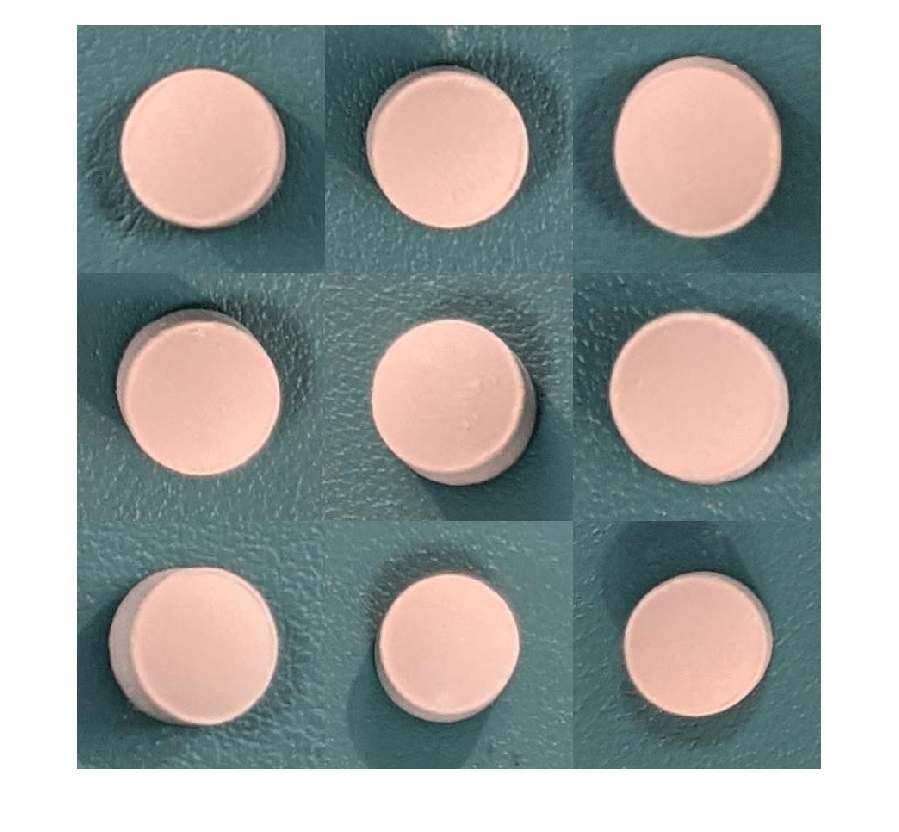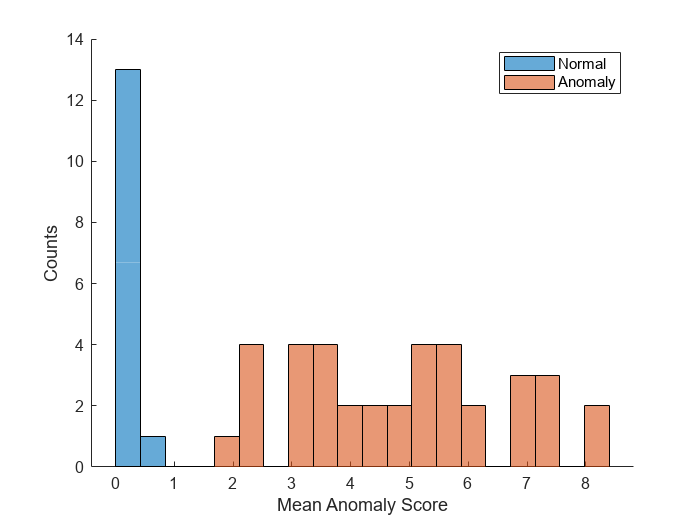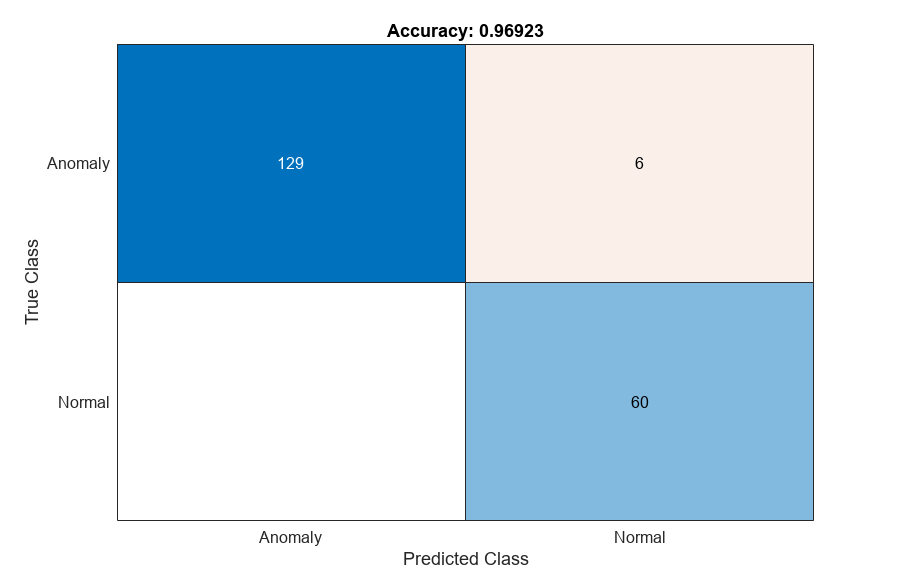# Detect Image Anomalies Using Explainable One-Class Classification Neural Network

This example shows how to train an anomaly detector for visual inspection of pill images.

In one-class anomaly detection approaches, training is semisupervised, meaning that the network trains on data consisting only of normal images without anomalies . Despite training on samples only of normal scenes, the model learns how to distinguish between normal and anomalous scenes. One-class learning offers many advantages for anomaly detection problems:

• Representations of anomalies can be scarce.

• Anomalies can represent expensive or catastrophic outcomes.

• There can be many kinds of anomalies and the kinds of anomalies can change over the lifetime of the model. Describing what "good" looks like is often more feasible than providing data that represents all possible anomalies in real world settings.

A crucial goal of anomaly detection is for a human observer to be able to understand why a trained network classifies images as anomalies. Explainable classification supplements the class prediction with information that justifies how the neural network reached its classification decision.

This example explores how one-class deep learning can be used to create effective anomaly detectors. The example also implements explainable classification using a network that returns a heatmap with the probability that each pixel is anomalous. The classifier labels images as normal or anomalous based on the mean value of the anomaly score heatmap.This example uses the pillQC data set. The data set contains images from three classes: `normal` images without defects, `chip` images with chip defects in the pills, and `dirt` images with dirt contamination. The data set provides 149 `normal` images, 43 `chip` images, and 138 `dirt` images. The size of the data set is 3.57 MB.

Set `dataDir` as the desired location of the data set. Download the data set using the `downloadPillQCData` helper function. This function is attached to the example as a supporting file. The function downloads a ZIP file and extracts the data into the subdirectories `chip`, `dirt`, and `normal`.

```dataDir = fullfile(tempdir,"PillDefects"); downloadPillQCData(dataDir)```
```Downloading Pill QC data set. This can take several minutes to download and unzip... Done. ```

This image shows an example image from each class. A normal pill with no defects is on the left, a pill contaminated with dirt is in the middle, and a pill with a chip defect is on the right. While the images in this data set contain instances of shadows, focus blurring, and background color variation, the approach used in this example is robust to these image acquisition artifacts.Create an `imageDatastore` that reads and manages the image data. Label each image as `chip`, `dirt`, or `normal` according to the name of its directory.

```imageDir = fullfile(dataDir,"pillQC-main","images"); imds = imageDatastore(imageDir,IncludeSubfolders=true,LabelSource="foldernames");```

#### Partition Data into Training, Calibration, and Test Sets

To simulate a more typical semisupervised workflow, create a training set of 70 images from the `normal` class. Include two anomalous training images from each of the `chip` and `dirt` classes to get better classification results. Allocate 30 normal images and 15 images from each anomalous class to a calibration set. This example uses a calibration set to pick a threshold for the classifier. The classifier labels images with anomaly scores above the threshold as anomalous. Using separate calibration and test sets avoids information leaking from the test set into the design of the classifier. Assign the remaining images to the test set.

```numTrainNormal = 70; numTrainAnomaly = 2; numCalNormal = 30; numCalAnomaly = 15; rng(0); [imdsNormalTrain,imdsNormalCal,imdsNormalTest] = splitEachLabel(imds,numTrainNormal,numCalNormal,"randomized",Include="normal"); [imdsAnomalyTrain,imdsAnomalyCal,imdsAnomalyTest] = splitEachLabel(imds,numTrainAnomaly,numCalAnomaly,"randomized",Include=["chip","dirt"]); imdsCal = imageDatastore(vertcat(imdsNormalCal.Files,imdsAnomalyCal.Files),LabelSource="foldernames",IncludeSubfolders=true); imdsTest = imageDatastore(vertcat(imdsNormalTest.Files,imdsAnomalyTest.Files),LabelSource="foldernames",IncludeSubfolders=true);```

#### Augment Training Data

Augment the training data by using the `transform` function with custom preprocessing operations specified by the helper function `augmentDataForPillAnomalyDetector`. The helper function is attached to the example as supporting files.

The `augmentDataForPillAnomalyDetector` function randomly applies 90 degree rotation and horizontal and vertical reflection to each input image.

```imdsNormalTrain = transform(imdsNormalTrain,@augmentDataForPillAnomalyDetector); imdsAnomalyTrain = transform(imdsAnomalyTrain,@augmentDataForPillAnomalyDetector);```

Add binary labels to the calibration and test data sets by using the `transform` function with the operations specified by the `addLabelData` helper function. The helper function is defined at the end of this example, and assigns images in the `normal` class a binary label `0` and images in the `chip` or `dirt` classes a binary label `1`.

```dsCal = transform(imdsCal,@addLabelData,IncludeInfo=true); dsTest = transform(imdsTest,@addLabelData,IncludeInfo=true);```

Visualize a sample of nine augmented training images.

```exampleData = readall(subset(imdsNormalTrain,1:9)); montage(exampleData(:,1));```### Create FCDD Model

This example uses a fully convolutional data description (FCDD) model . The basic idea of FCDD is to train a network to produce an anomaly score map that describes the probability that each region in the input image contains anomaly content.

The `pretrainedEncoderNetwork` function returns the first three downsampling stages of an ImageNet pretrained Inception-v3 network for use as a pretrained backbone.

`backbone = pretrainedEncoderNetwork("inceptionv3",3);`

Create an FCDD anomaly detector network by using the `fcddAnomalyDetector` (Computer Vision Toolbox) function with the Inception-v3 backbone.

`net = fcddAnomalyDetector(backbone);`

By default, this example downloads a pretrained version of the FCDD anomaly detector using the helper function `downloadTrainedNetwork`. The helper function is attached to this example as a supporting file. You can use the pretrained network to run the entire example without waiting for training to complete.

To train the network, set the `doTraining` variable in the following code to `true`. Specify the number of epochs to use for training `numEpochs` by entering a value in the field. Train the model by using the `trainFCDDAnomalyDetector` (Computer Vision Toolbox) function.

Train on one or more GPUs, if available. Using a GPU requires Parallel Computing Toolbox™ and a CUDA® enabled NVIDIA® GPU. For more information, see GPU Computing Requirements (Parallel Computing Toolbox). Training takes about 3 minutes on an NVIDIA Titan RTX™.

```doTraining =false; numEpochs =200; if doTraining options = trainingOptions("adam", ... Shuffle="every-epoch",... MaxEpochs=numEpochs,InitialLearnRate=1e-4, ... MiniBatchSize=32,... BatchNormalizationStatistics="moving"); detector = trainFCDDAnomalyDetector(imdsNormalTrain,imdsAnomalyTrain,net,options); modelDateTime = string(datetime("now",Format="yyyy-MM-dd-HH-mm-ss")); save(fullfile(dataDir,"trainedPillAnomalyDetector-"+modelDateTime+".mat"),"detector"); else trainedPillAnomalyDetectorNet_url = ... "https://ssd.mathworks.com/supportfiles/vision/data/trainedFCDDPillAnomalyDetectorSpkg.zip"; downloadTrainedNetwork(trainedPillAnomalyDetectorNet_url,dataDir); net = load(fullfile(dataDir,"folderForSupportFilesInceptionModel","trainedPillFCDDNet.mat")); detector = net.detector; end```
```Downloading pretrained network. This can take several minutes to download... Done. ```

### Set Anomaly Threshold

Select an anomaly score threshold for the anomaly detector, which classifies images based on whether their scores are above or below the threshold value. This example uses a calibration data set that contains both normal and anomalous images to select the threshold.

Obtain the mean anomaly score and ground truth label for each image in the calibration set.

```scores = predict(detector,dsCal); labels = imdsCal.Labels ~= "normal";```

Plot a histogram of the mean anomaly scores for the normal and anomaly classes. The distributions are well separated by the model-predicted anomaly score.

```numBins = 20; [~,edges] = histcounts(scores,numBins); figure hold on hNormal = histogram(scores(labels==0),edges); hAnomaly = histogram(scores(labels==1),edges); hold off legend([hNormal,hAnomaly],"Normal","Anomaly") xlabel("Mean Anomaly Score") ylabel("Counts")```Calculate the optimal anomaly threshold by using the `anomalyThreshold` (Computer Vision Toolbox) function. Specify the first two input arguments as the ground truth labels, `labels`, and predicted anomaly scores, `scores`, for the calibration data set. Specify the third input argument as `true` because true positive anomaly images have a `labels` value of `true`. The `anomalyThreshold` function returns the optimal threshold and the receiver operating characteristic (ROC) curve for the detector, stored as an `rocmetrics` (Deep Learning Toolbox) object.

`[thresh,roc] = anomalyThreshold(labels,scores,true);`

Set the `Threshold` property of the anomaly detector to the optimal value.

`detector.Threshold = thresh;`

Plot the ROC returned by `anomalyThreshold` by using the `plot` (Deep Learning Toolbox) object function of `rocmetrics`. The ROC curve illustrates the performance of the classifier for a range of possible threshold values. Each point on the ROC curve represents the false positive rate (x-coordinate) and true positive rate (y-coordinate) when the calibration set images are classified using a different threshold value. The solid blue line represents the ROC curve. The red dashed line represents a no-skill classifier corresponding to a 50% success rate. The ROC area under the curve (AUC) metric indicates classifier performance, and the maximum ROC AUC corresponding to a perfect classifier is 1.0.

```plot(roc) title("ROC AUC: "+ roc.AUC)```### Evaluate Classification Model

Classify each image in the test set as either normal or anomalous.

`testSetOutputLabels = classify(detector,dsTest);`

Get the ground truth labels of each test image.

`testSetTargetLabels = dsTest.UnderlyingDatastores{1}.Labels;`

Evaluate the anomaly detector by calculating performance metrics by using the `evaluateAnomalyDetection` (Computer Vision Toolbox) function. The function calculates several metrics that evaluate the accuracy, precision, sensitivity, and specificity of the detector for the test data set.

`metrics = evaluateAnomalyDetection(testSetOutputLabels,testSetTargetLabels,["dirt","chip"]);`
```Evaluating anomaly detection results ------------------------------------ * Finalizing... Done. * Data set metrics: GlobalAccuracy MeanAccuracy Precision Recall Specificity F1Score FalsePositiveRate FalseNegativeRate ______________ ____________ _________ ______ ___________ _______ _________________ _________________ 1 1 1 1 1 1 0 0 ```

The `ConfusionMatrix` property of `metrics` contains the confusion matrix for the test set. Extract the confusion matrix and display a confusion plot. The classification model in this example is very accurate and predicts a small percentage of false positives and false negatives.

```M = metrics.ConfusionMatrix{:,:}; confusionchart(M,["Normal","Anomaly"]) acc = sum(diag(M)) / sum(M,"all"); title("Accuracy: "+acc)```If you specify multiple anomaly class labels, such as `dirt` and `chip` in this example, the `evaluateAnomalyDetection` function calculates metrics for the whole data set and for each anomaly class. The per-class metrics are returned in the `ClassMetrics` property of the `anomalyDetectionMetrics` (Computer Vision Toolbox) object, `metrics`.

`metrics.ClassMetrics`
```ans=2×2 table Accuracy AccuracyPerSubClass ________ ___________________ Normal 1 {1×1 table} Anomaly 1 {2×1 table} ```
`metrics.ClassMetrics(2,"AccuracyPerSubClass").AccuracyPerSubClass{1}`
```ans=2×1 table AccuracyPerSubClass ___________________ chip 1 dirt 1 ```

### Explain Classification Decisions

You can use the anomaly heatmap predicted by the anomaly detector to help explain why an image is classified as normal or anomalous. This approach is useful for identifying patterns in false negatives and false positives. You can use these patterns to identify strategies for increasing class balancing of the training data or improving the network performance.

#### Calculate Anomaly Heat Map Display Range

Calculate a display range that reflects the range of anomaly scores observed across the entire calibration set, including normal and anomalous images. Using the same display range across images allows you to compare images more easily than if you scale each image to its own minimum and maximum. Apply the display range for all heatmaps in this example.

```minMapVal = inf; maxMapVal = -inf; reset(dsCal) while hasdata(dsCal) img = read(dsCal); map = anomalyMap(detector,img{1}); minMapVal = min(min(map,[],"all"),minMapVal); maxMapVal = max(max(map,[],"all"),maxMapVal); end displayRange = [minMapVal,maxMapVal];```

#### View Heatmap of Anomaly Image

Select an image of a correctly classified anomaly. This result is a true positive classification. Display the image.

```testSetAnomalyLabels = testSetTargetLabels ~= "normal"; idxTruePositive = find(testSetAnomalyLabels' & testSetOutputLabels,1,"last"); dsExample = subset(dsTest,idxTruePositive); img = read(dsExample); img = img{1}; map = anomalyMap(detector,img); imshow(anomalyMapOverlay(img,map,MapRange=displayRange,Blend="equal"))```#### View Heatmap of Normal Image

Select and display an image of a correctly classified normal image. This result is a true negative classification.

```idxTrueNegative = find(~(testSetAnomalyLabels' | testSetOutputLabels)); dsExample = subset(dsTest,idxTrueNegative); img = read(dsExample); img = img{1}; map = anomalyMap(detector,img); imshow(anomalyMapOverlay(img,map,MapRange=displayRange,Blend="equal"))```#### View Heatmaps of False Negative Images

False negatives are images with pill defect anomalies that the network classifies as normal. Use the explanation from the network to gain insights into the misclassifications.

Find any false negative images from the test set. Obtain heatmap overlays of the false negative images by using the `transform` function. The operations of the transform are specified by an anonymous function that applies the `anomalyMapOverlay` (Computer Vision Toolbox) function to obtain heatmap overlays for each false negative in the test set.

```falseNegativeIdx = find(testSetAnomalyLabels' & ~testSetOutputLabels); if ~isempty(falseNegativeIdx) fnExamples = subset(dsTest,falseNegativeIdx); fnExamplesWithHeatmapOverlays = transform(fnExamples,@(x) {anomalyMapOverlay(x{1},anomalyMap(detector,x{1}),MapRange=displayRange,Blend="equal")}); fnExamples = readall(fnExamples); fnExamples = fnExamples(:,1); fnExamplesWithHeatmapOverlays = readall(fnExamplesWithHeatmapOverlays); montage(fnExamples) montage(fnExamplesWithHeatmapOverlays) else disp("No false negatives detected.") end```
```No false negatives detected. ```

#### View Heatmaps of False Positive Images

False positives are images without pill defect anomalies that the network classifies as anomalous. Find any false positives in the test set. Use the explanation from the network to gain insights into the misclassifications. For example, if anomalous scores are localized to the image background, you can explore suppressing the background during preprocessing.

```falsePositiveIdx = find(~testSetAnomalyLabels' & testSetOutputLabels); if ~isempty(falsePositiveIdx) fpExamples = subset(dsTest,falsePositiveIdx); fpExamplesWithHeatmapOverlays = transform(fpExamples,@(x) {anomalyMapOverlay(x{1},anomalyMap(detector,x{1}),MapRange=displayRange,Blend="equal")}); fpExamples = readall(fpExamples); fpExamples = fpExamples(:,1); fpExamplesWithHeatmapOverlays = readall(fpExamplesWithHeatmapOverlays); montage(fpExamples) montage(fpExamplesWithHeatmapOverlays) else disp("No false positives detected.") end```
```No false positives detected. ```

### Supporting Functions

The `addLabelData` helper function creates a one-hot encoded representation of label information in `data`.

```function [data,info] = addLabelData(data,info) if info.Label == categorical("normal") onehotencoding = 0; else onehotencoding = 1; end data = {data,onehotencoding}; end```

### References

 Liznerski, Philipp, Lukas Ruff, Robert A. Vandermeulen, Billy Joe Franks, Marius Kloft, and Klaus-Robert Müller. "Explainable Deep One-Class Classification." Preprint, submitted March 18, 2021. https://arxiv.org/abs/2007.01760.

 Ruff, Lukas, Robert A. Vandermeulen, Billy Joe Franks, Klaus-Robert Müller, and Marius Kloft. "Rethinking Assumptions in Deep Anomaly Detection." Preprint, submitted May 30, 2020. https://arxiv.org/abs/2006.00339.

 Simonyan, Karen, and Andrew Zisserman. "Very Deep Convolutional Networks for Large-Scale Image Recognition." Preprint, submitted April 10, 2015. https://arxiv.org/abs/1409.1556.

 ImageNet. https://www.image-net.org.